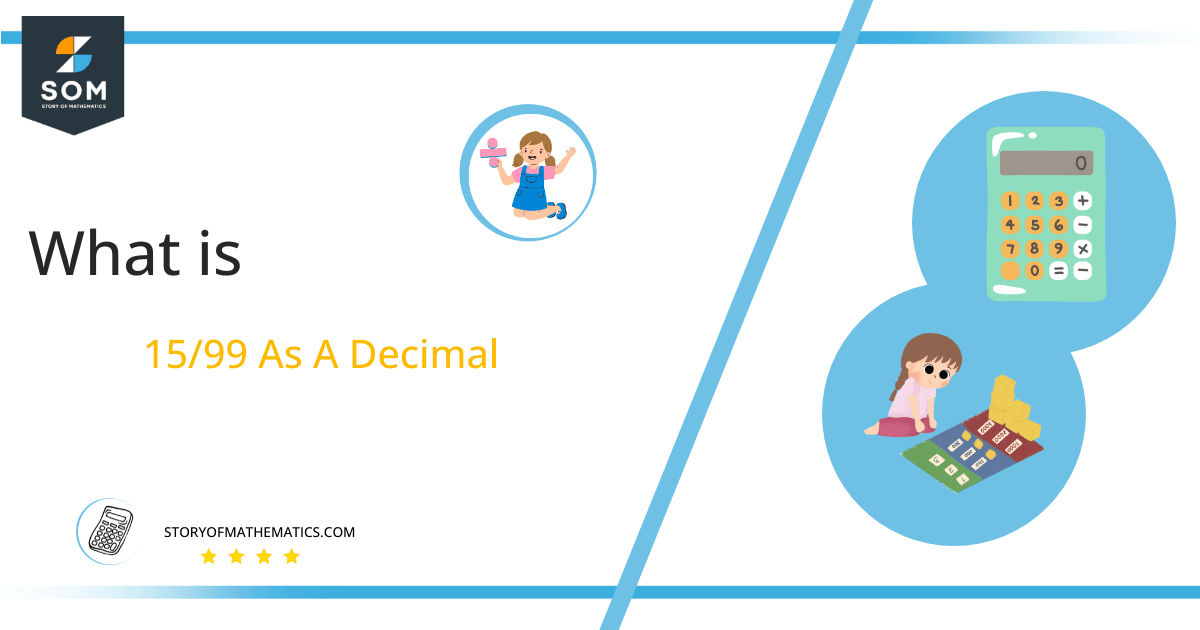# What Is 15/99 as a Decimal + Solution With Free Steps

The fraction 15/99 as a decimal is equal to 0.151.

We know that division is one of the four main mathematical operators, and there are two kinds of divisions. One solves completely and yields an Integer value, whereas the other does not translate to completion and yields a Decimal value.

Here, we are more interested in the division types that result in a Decimal value, as this can be expressed as a Fraction. We see fractions as a way of showing two numbers having the operation of Division between them that result in a value that lies between two Integers.Now, we introduce the method used to solve said fraction to decimal conversion, called Long Division, which we will discuss in detail moving forward. So, let’s go through the Solution of fraction 15/99. Figure 1 shows how long division is done:Figure 1

## Solution

First, we convert the fraction components, i.e., the numerator and the denominator, and transform them into the division constituents, i.e., the Dividend and the Divisor, respectively.

This can be done as follows:

Dividend = 15

Divisor = 99

Now, we introduce the most important quantity in our division process: the Quotient. The value represents the Solution to our division and can be expressed as having the following relationship with the Division constituents:

Quotient = Dividend $\div$ Divisor = 15 $\div$ 99

This is when we go through the Long Division solution to our problem.

## 15/99 Long Division Method

We start solving a problem using the Long Division Method by first taking apart the division’s components and comparing them. As we have 15 and 99, we can see how 15 is Smaller than 99 and to solve this division, we require that 15 be Bigger than 99.

This is done by multiplying the dividend by 10 and checking whether it is bigger than the divisor or not. If so, we calculate the Multiple of the divisor closest to the dividend and subtract it from the Dividend. This produces the Remainder, which we then use as the dividend later.

Now, we begin solving for our dividend 150, which after getting multiplied by 10 becomes 150.

We take this 150 and divide it by 99; this can be done as follows:

150 $\div$ 99 $\approx$ 1

Where:

99 x 1 = 99

This will lead to the generation of a Remainder equal to 150 – 99 = 51. Now this means we have to repeat the process by Converting the 51 into 510 and solving for that:

510 $\div$ 99 $\approx$ 5

Where:

99 x 5 = 495

This, therefore, produces another Remainder which is equal to 510 – 495 = 15. Now we must solve this problem to Third Decimal Place for accuracy, so we repeat the process with dividend 150.

150 $\div$ 99 $\approx$ 1

Where:

99 x 1 = 99

Finally, we have a Quotient generated after combining the three pieces of it as 0.515=z, with a Remainder equal to 51.Images/mathematical drawings are created with GeoGebra.